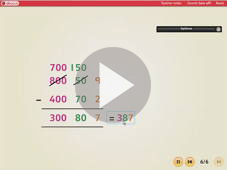Expanded written subtraction Worksheet Answers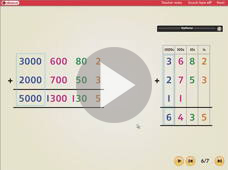Adding numbers using a standrad written method Worksheet Answers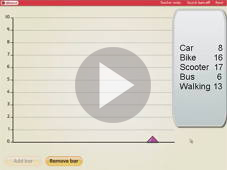Using a bar chart to show information Worksheet Answers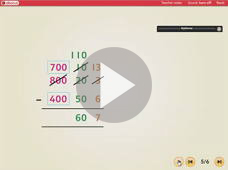Expanded written subtraction (part 2) Worksheet Answers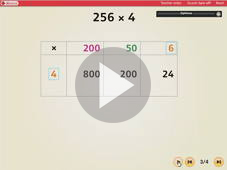Grid multiplication Worksheet Answers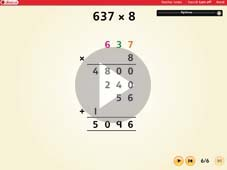Using the ladder method Worksheet Answers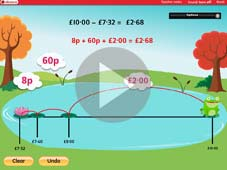Counting up to find change fro £10, £20 or £50 Worksheet Answers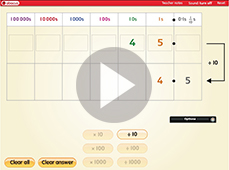Multiplying and dividing by 10 and 100 Worksheet Answers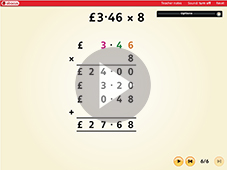Using the ladder method to multiply amounts of money Worksheet Answers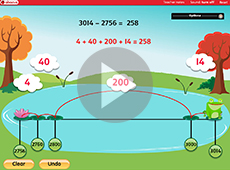Counting up to find a difference Worksheet Answers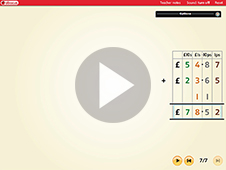Adding amounts of money using compact column method Worksheet Answers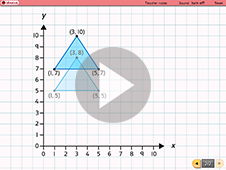Using co-ordinates to draw and translate polygons Worksheet Answers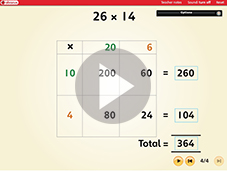Using the grid method to multiply a pair of 2 digit numbers. Worksheet Answers